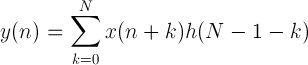# Convolution calculator

 Enter first data sequence: 1 1 1 0 0 0 Enter second data sequence: 0.5 0.2 0.3 Result data sequence:

## Convolution calculation

The sequence y(n) is equal to the convolution of sequences x(n) and h(n):For finite sequences x(n) with M values and h(n) with N values:For n = 0 .. M+N-2

### Convolution Calculator

convolution calculator combines two data sequence into a single data sequence. Enter the data sequences into its appropriate position and click on calculate to get unique single data sequence.Convolution is a mathematical operation, which applies on two values say X and H and gives a third value as an output say Y.

In convolution, we do point to point multiplication of input functions and gets our output function.

Convolution function:

A mathematical function performs on two functions to produce the third function. Convolution is a combination of result function and computing process.

If convolution applied on two functions x(n), h(n) then the result function is y(n), then y(n) = x(n)*h(n).

Convolution Formulas:

The sequence y(n) is equal to the convolution of sequences x(n) and h(n):

y(n) = x(n)*h(n) = ∑ x(k)h(n-k)

For finite sequences x(n) with M values and h(n) with N values.

y(n) = ∑ x(n+k)h(N-1-K) where n = 0 .. M+N-2

How to use the convolution calculator?

To use our calculator you need to follow below steps.

1. Open convolution calculator.

2. Enter the two data sequence or functions.

3. Click on calculate to get the third sequence